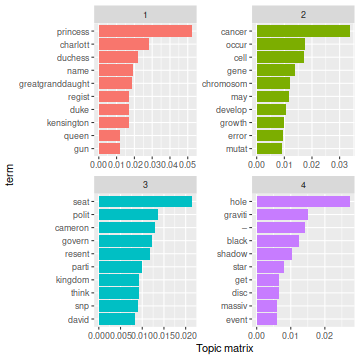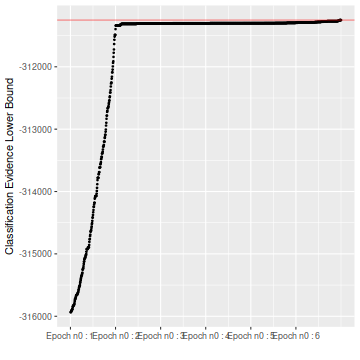set.seed(42)
library(MoMPCA)
library(aricode)

Description

MMPCA is a package to perform clustering of count data based on the mixture of multinomial PCA model. It integrates a dimension reduction aspect by factorizing the multinomial parameters in a latent space, like Latent Dirichlet Allocation of Blei et. al. It specially conceived for low sample high-dimensional data. Due to the intensive nature of the greedy algorithm, it is not suited for large sample size.

Dataset

The package contains attached data in BBCmsg. It consists in 4 text document already preprocessed with the tm package. It is mostly useful for the simulate_BBC() function.

data("BBCmsg")

Demonstration for document clustering

Start by generating data from the MMPCA model with a particular $$\theta^\star$$ and $$\beta^\star$$. For more detail, check experimental section of the paper.

N = 200
L = 250
simu <- simulate_BBC(N, L, epsilon = 0, lambda = 1)
Ytruth <- simu$Ytruth Then perform clustering t0 <- system.time(res <- mmpca_clust(simu$dtm.full, Q = 6, K = 4,
Yinit = 'random',
method = 'BBCVEM',
max.epochs = 7,
keep = 1,
verbose = 2,
nruns = 2,
mc.cores = 2)
)
print(t0)
#>    user  system elapsed
#>   0.035   0.005 100.248

Results analysis

tab <- knitr::kable(table(res@clustering, Ytruth), format = 'markdown')
print(tab)
#>
#>
#> |  1|  2|  3|  4|  5|  6|
#> |--:|--:|--:|--:|--:|--:|
#> | 34|  0|  0|  0|  0|  0|
#> |  0|  1|  0|  1|  0| 37|
#> |  0|  0|  0| 35|  0|  0|
#> |  2|  0|  0|  0| 28|  0|
#> |  0|  0| 26|  0|  4|  0|
#> |  0| 32|  0|  0|  0|  0|
cat('Final ARI is ', aricode::ARI(res@clustering, Ytruth))
#> Final ARI is  0.9117862

Other visualization are also accessible from the plot function. Which takes several arguments

ggtopics <- plot(res, type = 'topics')
print(ggtopics)ggbound <- plot(res, type = 'bound')
print(ggbound)Model selection

The package contains a convenient wrapper around mmpca_clust() which performs model selection over a grid of values for $$(K,Q)$$. Here is the results for Qs = 5:7 and Ks = 3:5.

t1 <- system.time(res <- mmpca_clust_modelselect(simu$dtm.full, Qs = 5:7, Ks = 3:5, Yinit = 'kmeans_lda', init.beta = 'lda', method = 'BBCVEM', max.epochs = 7, nruns = 3, verbose = 1) ) print(t1) best_model = res$models
print(best_model)